## Quantum bits

• Computers provide information through units known as bits:
• Bits are likely stored as tiny electric charges on nanometer-scale capacitors;
• A bit can have a state of either 1 or 0;
• Everything a computer does can be broken down into a combination of 1’s and 0’s.
• Similarly, quantum computers use quantum bits, or qubits:
• Just like a bit, qubits have a state;
• Whereas the state of a bit is a number (0 or 1), the state of a qubit is a vector;
• Specifically, the state of a qubit is a vector in a two-dimensional vector space;
• This vector space is known as state space.

$$\begin{bmatrix} 1\\0 \end{bmatrix}$$

Possible qubit state

### Review:

1. The quantum equivalent of a bit is a qubit;
2. Qubits have a state;
3. Much like a bit, that state is an abstract mathematical object;
4. Whereas a bit’s abstract state is a number, 0 or 1, the state of a qubit is a 2-dimensional vector;
5. We call the 2-dimensional vector space where states live state space.

Henceforth we’ll use the phrase “classical bit”, after “classical physics” to describe the informational unit we are familiar with in ordinary computing.

## Computational basis states

There are two special quantum states which correspond to the $$0$$ and the $$1$$ states of a classical bit. The quantum state corresponding to $$0$$ is usually denoted $$\mid0 \rangle$$. That’s notation for the following vector:

$$\mid0 \rangle:=\begin{bmatrix} 1\\0 \end{bmatrix}$$

A computational basis state

This notation with $$\mid$$ and $$\rangle$$ is called the ket notation, and things like $$\mid0 \rangle$$ are called kets. Don’t be thrown off by the unfamiliar terminology – a ket is just a vector, and when we say something is a ket, all we mean is that it’s a vector.

• |0⟩ is a computational basis state for a qubit, and plays much the same role as 0 does for a classical bit
• There is a another computational basis state, denoted $$\mid1 \rangle$$ , which plays the same role as 1 does for a bit
• Like $$\mid0 \rangle$$, $$\mid1 \rangle$$ is just a notation for a two-dimensional vector, in this case:

$$\mid 1 \rangle:=\begin{bmatrix} 0\\1 \end{bmatrix}$$

Another computational basis state

## General qubit states

The computational basis states $$\mid0 \rangle$$ and $$\mid1 \rangle$$ are just two possible states for a qubit. Many more states are possible, and those extra states endow qubits with capabilities not available to ordinary classical bits. In general, remember, a quantum state is a two-dimensional vector. Here’s an example, with a graphical illustration emphasizing the vector nature of the state:Example vector state

The state $$0.6 \mid 0 \rangle+0.8 \mid 1 \rangle$$ is just 0.6 times the $$\mid 0 \rangle$$ vector, plus 0.8 times the $$\mid 1 \rangle$$ vector. In the usual vector notation that means the state is:

$$0.6 \mid 0 \rangle + 0.8 \mid 1 \rangle=0.6 \begin{bmatrix} 1\\0 \end{bmatrix} + 0.8 \begin{bmatrix} 0\\1 \end{bmatrix} = \begin{bmatrix} 0.6\\0.8 \end{bmatrix}$$

Quantum states are not only two-dimensional vectors, they are complex vectors, that is, they can have complex numbers as entries. So, for instance, another quantum vector is the state:

$$\frac{1+i}{2}\mid 0 \rangle + \frac{i}{\sqrt{2}}\mid 1 \rangle,$$

which can be written in the conventional component vector representation as:

$$\begin{bmatrix} \frac{1+i}{2}\\\frac{i}{\sqrt{2}} \end{bmatrix}$$

## Common terminology

People will say a state like $$0.6 \mid 0 \rangle+0.8 \mid 1 \rangle$$ is a superposition of $$\mid0 \rangle$$ and $$\mid1 \rangle$$. All they mean is that the state is a linear combination of $$\mid0 \rangle$$ and $$\mid1 \rangle$$.

Another common term is amplitude. An amplitude is the coefficient for a particular state in superposition. For instance, in the state $$0.6 \mid 0 \rangle+0.8 \mid 1 \rangle$$ the amplitude for $$\mid0 \rangle$$ is 0.6 and the amplitude for $$\mid1 \rangle$$ is 0.8.

## Normalisation constraint

Recall that a quantum state is a two-dimensional complex vector. Actually, it can’t be just any vector—there’s a constraint. The constraint is this: the sums of the squares of the amplitudes must be equal to 1.

For example, for the state $$0.6 \mid 0 \rangle+0.8 \mid 1 \rangle$$ the sum of the squares of the amplitudes is $$0.6^2+0.8^2$$, which is $$0.36+0.64=1$$, as we desired.

In more general states, the amplitudes can be complex numbers denoted by alpha $$(\alpha)$$ and beta $$(\beta)$$. The constraint is now that the sum of the squares of the amplitudes is 1. This is called the normalization constraint:

$$\mid \alpha^2\mid + \mid \beta^2\mid = 1$$

The normalisation constraint

It’s called that because if you think of $$\mid0 \rangle$$ and $$\mid1 \rangle$$ as orthonormal vectors, like this:A normalised vector, visualised

Then the normalization constraint is the requirement that the length of the state is equal to 1. So it’s a unit vector, or a normalized vector, and that’s why this is called a normalization constraint.

The quantum state of a qubit is a vector of unit length in a two-dimensional complex vector space known as state space.

## Quantum logic gates

In a classical NOT logic gate, the input and output are swapped. An input of $$1$$ becomes an output of $$0$$, etc.

On a computational basis, the quantum NOT gate does the same:

$$NOT \mid 0 \rangle = \mid 1 \rangle$$ $$NOT \mid 1 \rangle = \mid 0 \rangle$$

The computational basis states aren’t the only states possible for a qubit. When a NOT gate is applied to a general superposition state, that is, $$\alpha \mid 0 \rangle + \beta \mid 1 \rangle$$, it acts linearly on the quantum state—interchanging the role of $$\mid0 \rangle$$ and $$\mid1 \rangle$$:

$$NOT(\alpha \mid 0 + \beta \mid 1 \rangle) = \alpha \mid 1 \rangle + \beta \mid 0 \rangle$$

NOT gates are usually denoted by the symbol $$X$$, so the above may be rewritten:

$$X(\alpha \mid 0 + \beta \mid 1 \rangle) = \alpha \mid 1 \rangle + \beta \mid 0 \rangle$$

## Quantum circuits

So far we have seen algebraic ways of writing down the way the $$X$$ gate works. There’s an alternate representation, the quantum circuit representation. In a quantum circuit we depict an $$X$$ gate as follows: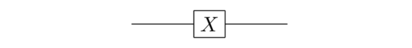A quantum circuit w/ X gate

The line from left to right is what’s called a quantum wire. A quantum wire represents a single qubit. The term “wire” and the way it’s drawn looks like the qubit is moving through space. But it's often helpful to instead think of left-to-right as representing the passage of time. So the initial segment of wire is just the passage of time, with nothing happening to the qubit. Then the $$X$$ gate is applied. And then the quantum wire continues, leaving the desired output.

Sometimes we’ll put the input and output states explicitly in the quantum circuit, so we have something like: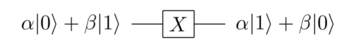Quantum circuit representation of the X gate

This may also be represented as a $$2 \times 2$$ matrix:

$$X = \begin{bmatrix} 0 & 1\\ 1 & 0 \end{bmatrix}$$

## Multi-gate quantum circuits

A simple multi-gate quantum circuit is shown below. It’s just a circuit with two $$X$$ gates in a row: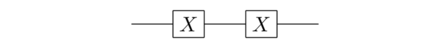A simple multi-gate circuit

Visualising this in a matrix representation:

$$XX = \begin{bmatrix} 0 & 1\\ 1 & 0 \end{bmatrix} \begin{bmatrix} 0 & 1\\ 1 & 0 \end{bmatrix} = \begin{bmatrix} 1 & 0\\ 0 & 1 \end{bmatrix}$$

Thus, this circuit would be equivalent to a quantum wire: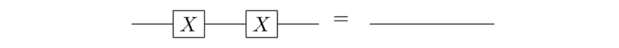Multi-gate equivalence

The first quantum gate, the $$NOT$$ or $$X$$ gate doesn’t do much more than what is possible with a classical NOT gate. Let’s explore the Hadamard gate. Denoting the gate by $$H$$:

$$H \mid 0 \rangle = \frac{\mid 0 \rangle + \mid 1 \rangle}{\sqrt{2}}$$ $$H \mid 1 \rangle = \frac{\mid 0 \rangle - \mid 1 \rangle}{\sqrt{2}}$$

Just like the $$X$$ gate, $$H$$ has a matrix representation:

$$H = \frac{1}{\sqrt{2}} \begin{bmatrix} 1 & 1\\1 & -1 \end{bmatrix}$$

## Measuring a qubit

• The quantum state of any system is not directly observable
• There is no way to figure out the identity of $$\alpha$$ or $$\beta$$ if they start out unknown
• The amplitudes are kind of a hidden information
• Rather than somehow measuring these amplitudes, we can extract information by a process called measurement in the computational basis
• If we measure a qubit with state $$\alpha \mid 0 \rangle + \beta \mid 1 \rangle$$ in the computational basis, then the outcome is a classical bit
• Either $$0$$, with a probability $$\mid \alpha \mid^2$$
• Or $$1$$, with a probability $$\mid \beta \mid ^2$$
• The corresponding state of the qubit after the measurement is $$\mid0 \rangle$$ or $$\mid1 \rangle$$
• After the the measurement, no matter what the outcome, $$\alpha$$ and $$\beta$$ are gone
• This means you cannot store an infinite amount of classical information in a qubit

So the way a quantum computation works is that we manipulate a quantum state using a series of quantum gates, and then at the end of the computation (typically) we do a measurement to read out the result of the computation. If our quantum computer is just a single qubit, then that result will be a single classical bit. If, as is more usually the case, it’s multiple qubits, then the measurement result will be multiple classical bits.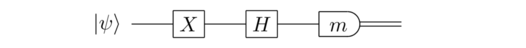Example measurement denotation

It’s a single-qubit quantum circuit, with input the state $$\mid \psi \rangle$$. A $$NOT$$ gate is applied, followed by a Hadamard gate. The circuit finishes with a measurement in the computational basis, denoted by the slightly elongated semi-circle. The $$m$$ is a classical bit denoting the measurement result – either $$0$$ or $$1$$ – and we use the double wire to indicate the classical bit $$m$$ going off and being used to do something else.

## General single-qubit gates

We now know about the NOT and Hadamard gates, and also about the measurement process that can be used to extract classical information from our quantum circuits. In this section, let’s return to quantum gates, and take a look at the most general single-qubit gate. To do that it helps to recall the matrix representations of the NOT and Hadamard gates:

$$X = \begin{bmatrix} 0 & 1\\ 1 & 0 \end{bmatrix}; H= \frac{1}{\sqrt{2}} \begin{bmatrix} 1 & 1\\ 1 & -1 \end{bmatrix}$$

If the input to these gates is the quantum state $$\mid \psi \rangle$$, then the output is $$X \mid \psi \rangle$$ and $$H \mid \psi \rangle$$ respectively.

A general single-qubit gate works similarly. In particular, a general single-qubit gate can be represented as a $$2 \times 2$$ unitary matrix, U. If the input to the gate is the state $$\mid \psi \rangle$$ then the output from the gate is $$U \mid \psi \rangle$$. And so the NOT and Hadamard gates correspond to the special cases where $$U = X$$ and $$U = H$$, respectively.

What does it mean for a matrix $$U$$ to be unitary? It’s easiest to answer this question algebraically, where it simply means that $$U^{\dagger}U=I$$, that is, the adjoint of $$u$$, denoted $$U^{\dagger}$$, times $$U$$, is equal to the identity matrix. That adjoint is, recall, the complex transpose of $$U$$:

$$U^{\dagger}:=(U^{T})*$$

So for a $$2 \times 2$$ matrix, the adjoint operation is just:

$$\begin{bmatrix} a & b\\c & d \end{bmatrix}^\dagger = \begin{bmatrix} a^* & c^*\\b^* & d^* \end{bmatrix}$$

The $$\dagger$$ is also sometimes called the dagger operation, or *Hermitian conjugation, or just the conjugation operation. We’ll use all three terms on occasion.

As well as the $$X$$ gate already introduced, there are $$Y$$ and $$Z$$ gates which, combined with the $$X$$ gates, are collectively known as the Pauli matrices. These are useful tools in our quantum gate toolkit and they expand the repertoire of moves we have available to us. They’re crucial, for example, in protocols such as quantum teleportation and quantum error correction.

$$Y:=\begin{bmatrix} 0 & i\\i & 0 \end{bmatrix}$$ $$Z:=\begin{bmatrix} 1 & 0\\0 & -1 \end{bmatrix}$$

Another good example of a quantum gate is a rotation, the kind of matrix you’ve most likely been seeing since high school. It’s just the ordinary rotation of the 2-dimensional plane by an angle $$\theta$$

$$\begin{bmatrix} cos(\theta) & -sin(\theta)\\sin(\theta) & cos(\theta) \end{bmatrix}$$

## Unitary matrices

• Unitary matrices preserve the length of their inputs
• If we take any vector $$\mid \psi \rangle$$ and compute the length $$\mid \mid U \mid \psi \rangle \mid \mid$$ it’s always equal to the length $$\mid \mid \mid \psi \rangle \mid \mid$$ of the original vector
• They’re much like rotations or reflections in ordinary (real) space, which also don’t change lengths
• In a sense, the unitary matrices are a complex generalization of real rotations and reflections
• This is good for qunatum gates because the input state will be normalized (have length 1), since that’s a requirement for a quantum state
• Fortunately, the length-preserving property of unitary matrices ensures the output state is properly normalized

Not only are gates unitary, to ensure that states remain normalized; also, quantum states are normalized, since measurement probabilities must sum to 1. It all fits together. In fact, it turns out that unitary matrices are the only matrices which preserve length in this way. And so a good way of thinking about unitary matrices is that they’re exactly the class of matrices which preserve length. That’s the geometric interpretation (and intuitive meaning) of the algebraic condition $$U^{\dagger}U=I$$

## The controlled-NOT gate

We’ve developed most of the ideas needed to do universal quantum computing. We understand qubits, quantum states, and have a repertoire of quantum gates. However, all our gates involve just a single qubit. To compute, we need some way for qubits to interact with one another. That is, we need quantum gates which involve two (or more) qubits.

An example of such a gate is the controlled-NOT (or CNOT) gate. In the quantum circuit language we have two wires, representing two qubits, and the following notation to represent the CNOT gate:The CNOT gate

• The top wire with the small, filled dot is called the control qubit
• The bottom wire with the larger, unfilled circle on it is called the target qubit

The four computational basis states, corresponding to the four possible states of a two-bit system, are:

$$\mid 00 \rangle, \mid 01 \rangle, \mid 10 \rangle, \mid 11 \rangle$$

And, for a two-qubit system, not only can we have those four states, we can also have superpositions (i.e., linear combinations) of them:

$$\alpha \mid 00 \rangle + \beta \mid 01 \rangle + \gamma \mid 10 \rangle + \delta \mid 11 \rangle$$

The amplitudes $$\alpha, \beta, \gamma, \delta$$ are just complex numbers, and the sum of the squares of the absolute values is 1. This is the same kind of normalization condition as we had for a single qubit.

The CNOT gate operates very simply. If the control qubit is set to 1, as in the states $$\mid10\rangle$$ and $$\mid11\rangle$$, then it flips (i.e., NOTs) the target qubit. And otherwise it does nothing. Writing out the action on all four computational basis states we have:

$$\mid00\rangle \rightarrow \mid00\rangle$$ $$\mid01\rangle \rightarrow \mid01\rangle$$ $$\mid10\rangle \rightarrow \mid11\rangle$$ $$\mid11\rangle \rightarrow \mid10\rangle$$

The CNOT can be thought of as a very simple kind of if-then statement: if the control qubit is set, then NOT the target qubit. But while simple, it can be used as a building block to build up other, more complex kinds of conditional behavior.

Summing all four equations above into a single equation, suppose $$x$$ and $$y$$ are classical bits, i.e., 0 or 1. Then we can rewrite the rewrite the equations above in a single equation as:

$$\mid x,y \rangle \rightarrow \mid x,y \oplus x \rangle$$

The above equation makes clear that the CNOT leaves the control qubit $$x$$ alone, but flips the target qubit $$y$$ is $$x$$ is set to 1. Note that $$\oplus$$ is addition modulo 2, where $$1 \oplus 1 = 0$$, as we would expect from the fact that the CNOT takes $$\mid 11 \rangle$$ to $$\mid 10 \rangle$$. It is also worth noting that the CNOT is unitary, and thus preserves the length of quantum states, as we expect.

Of course, the CNOT doesn’t just appear in two-qubit computations. It also appears in computations involving more qubits. Let’s suppose we have three qubits, for instance, and computational basis states such as $$\mid 000 \rangle$$, $$\mid 001 \rangle$$, and so on. Here’s a CNOT with the second qubit as the control qubit and the third qubit as the target:Three-qubit computation diagram

What goes on? Well, we can write out what happens on an arbitrary computational basis state, $$\mid x,y,x \rangle$$, where $$x, y$$ and $$z$$ are all classical bits. Of course, the first bit $$x$$ isn’t changed at all, since it’s not involved in the CNOT. The second bit $$y$$ is the control bit, and so isn’t changed. But the third bit $$z$$ is flipped if the control bit $$y$$ is set to 1. And so we can write the action of the CNOT as:

$$\mid x,y,x \rangle \rightarrow \mid x,y,z \oplus y \rangle$$

CNOT has been described as a “classical” gate, but it can be combined with single-qubit gates to do non-classical things. An example is the diagram below, a two-qubit computation starting with the $$\mid 00 \rangle$$computational basis state and applying a Hadamard gate to the first qubit, and then do a CNOT:Non-classical example

Recall that for a single qubit the Hadamard gate takes $$\mid 0 \rangle$$ to an equal superposition $$(\mid 0 \rangle + \mid 1 \rangle) / \sqrt{2}$$. For those two qubits it doesn’t affect the second qubit at all, and so it takes $$\mid 00 \rangle$$ to $$(\mid 10 \rangle)/ \sqrt{2}$$.

Next we apply the CNOT gate. This leaves the $$\mid 00 \rangle$$ state unchanged, since the control bit is 0. And it takes $$\mid 10 \rangle$$ to $$\mid 11 \rangle$$, since the control bit is 1. And so the output from the circuit is:

$$\frac{\mid 00 \rangle + \mid 11 \rangle}{\sqrt{2}}$$

This output state is a highly non-classical state – it’s actually a type of state called an entangled state. There’s no obvious interpretation of this state as a classical state, unlike say a computational basis state such as $$\mid 00 \rangle$$. In fact, entangled states can be used to do all sorts of interesting information processing tasks, including quantum teleportation and fast quantum algorithms.

## Quantum computing summary

We can summarize the three steps in a quantum computation as follows:

1. Start in a computational basis state
2. Apply a sequence of CNOT and single-qubit gates
3. To obtain the result, measure in the computational basis. The probability of any result, say 00...0, is just the square of the absolute value of the corresponding amplitude

That’s all a general quantum computation is! If you understand this model, you know what a quantum computer is.